## Example Questions

### Example Question #14 : Solid Geometry

Quantity A: The length of a side of a cube with a volume of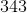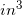.

Quantity B: The length of a side of a cube with surface area of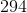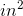.

Which of the following is true?

The two quantities are equal

Quantity B is larger.

The relationship between the two quantities cannot be determined.

Quantity A is larger.

The two quantities are equal

Explanation:

Recall that the equation for the volume of a cube is: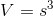Since the sides of a cube are merely squares, the surface area equation is justtimes the area of one of those squares: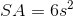So, for our two quantities:

Quantity A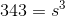Use your calculator to estimate this value (since you will not have a square root key). This is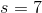.

Quantity B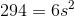First divide by: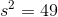Therefore,Therefore, the two quantities are equal.

### Example Question #15 : Solid Geometry

What is the length of an edge of a cube with a surface area of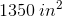?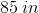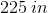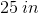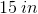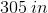Explanation:

The surface area of a cube is made up ofsquares. Therefore, the equation is merelytimes the area of one of those squares.  Since the sides of a square are equal, this is:, whereis the length of one side of the square.

For our data, we know: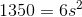This means that: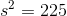Now, while you will not have a calculator with a square root key, you do know that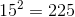. (You can always use your calculator to test values like this.) Therefore, we know that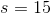. This is the length of one side

### Example Question #16 : Solid Geometry

If a cube has a total surface area ofsquare inches, what is the length of one edge?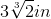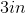There is not enough information given.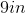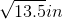Explanation:

There are 6 sides to a cube. If the total surface area is 54 square inches, then each face must have an area of 9 square inches.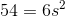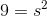Every face of a cube is a square, so if the area is 9 square inches, each edge must be 3 inches.

Tired of practice problems?

Try live online GRE prep today.